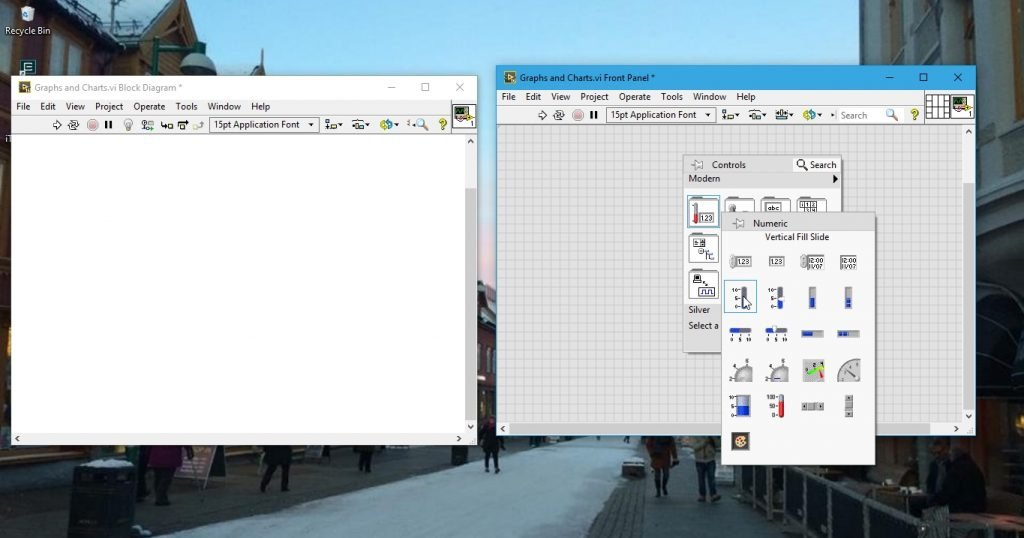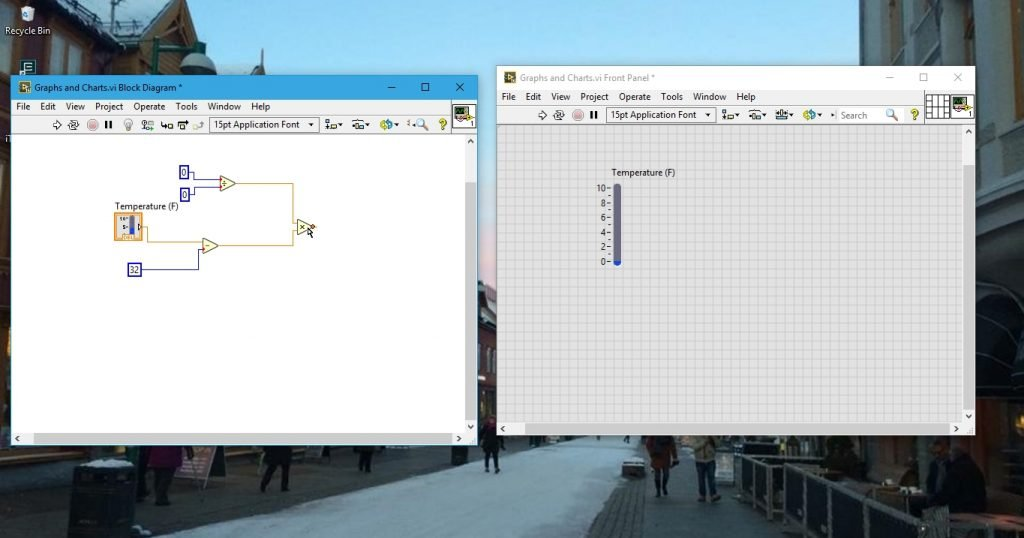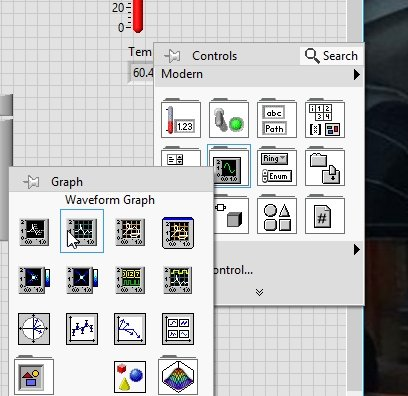# Using charts and graphs in labview with example: tutorial 6

In the tutorial we will focus on using charts, graphs fill slides and thermometers. First is the introduction and uses of graphs and charts then in the example we focused on, is about the conversion of temperature from Fahrenheit scale to Celsius scale using the formula of conversion we have been studying from elementary classes. The example program is explained point by point and the reference figures are added wherever required. At the end of the tutorial you are provided with an exercise which you are supposed to do on your own and in the upcoming tutorials I assume that you have done that example correctly and I am not going to explain the concepts regarding that exercise.

### About charts and graphs in labview

Someone said, “A picture is worth a thousand words”. What about graphs and charts??? Graphs and charts are always been helpful in data analysis and to see the variation of data with time. One can easily see the variation of data with time or any other independent variable when it is stored in the form of graphs or charts. There are various types of graphs and chart. Reading off information about the number of a particular thing in a category can also be done using charts or graphs without going back to the data table (which is quite a hideous task to perform). A graph can also be defined as a pictorial representation of data. The basic purpose of this tutorial is to familiarize the reader with the concepts of drawing graphs and charts in LabView programming.

In the previous tutorials we have learned about the introductions of LabView and learned to write a labview simple basic program to do string and numerical operations. In this tutorial we will use the built in graph and charts functionality blocks to plot various data points and plot the graphs.

### labview Programs including graphs and charts

• Create a new Blank VI as explained in the 1st tutorial and save it for future use as we have done in previous tutorials or press <Ctrl+S>
• After saving, come to the plotting part. Let’s begin with the introduction of simple functionality blocks.
• In the example below I am going to show you how charts and graphs work in LabView. I am going to design a simple LabView program that will convert temperature from Fahrenheit to Celsius scale; at the end of the conversion the VI will display the temperature variations on charts (colored scales).
• Right click on the front panel and from the control palette select numeric and then select Vertical slide bar. Name every block properly, this block will provide the temperature in Fahrenheit to be converted in Celsius.Figure 1: Vertical slide fill

• Using the formula for the conversion of temperature from one scale into other as given in the equation below:

Tc = 5/9(Tf – 32)

• To fulfill the conditions in the above equation we need division subtraction and multiplications block. Connect the output of the Temperature slider to in upper input of the subtraction block which is available in the function palette in numeric section, and at the other side of the subtraction block connect a numeric constant and set its value to 32.
• This task is accomplished in order to cover the portion (  of the equation defined above. From the same section select the division block and at the upper end of it connect a numeric constant of value 5 whereas at the lower input connect a numeric constant of value 9.
• Now, place a multiplier block from the same section at the input of which connect the output from the subtraction block and the division block we have just installed The resulting block diagram will look like the one shown in the figure below.Figure 2: Equation solution block diagram

Figure 3: Thermometer

Figure 4: Complete diagram for scale conversion

Figure 5: Diagram after indicators

Figure 6: Changing upper limit of the vertical slide fill

Figure 7: After running the VI

Figure 8: Waveform charts

Figure 9: Output of the waveform chart

### Graphs in labview

• Now, let’s come to the graphs portion. In order to see a graph of a data we have to have a data set of any type over some period of time. For that purpose we will use a ‘for’ loop set its number of iteration to a desired value and also place a wait block inside the loop, and place all of these blocks already present on the block diagram window inside the loop. From the output of the multiply block connect a wire to the edge of the ‘for’ loop, this will allow you to connect a block outside the loop. From the control palette select graphs and then select waveform graphs as shown below.Figure 10: Waveform graphs block

Figure 11: Graph block diagram

Figure 12: Final graph and chart output

From the above example we can conclude that the waveform charts in LabView gives us point to point information about the data with respect to time, whereas, the graphs gives us the information about the data variation on a defined period of time.

Exercise:

• Do the above example, and try to convert temperature from Celsius to Fahrenheit scale.